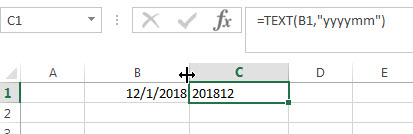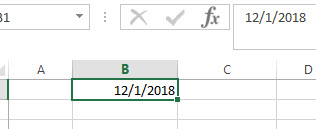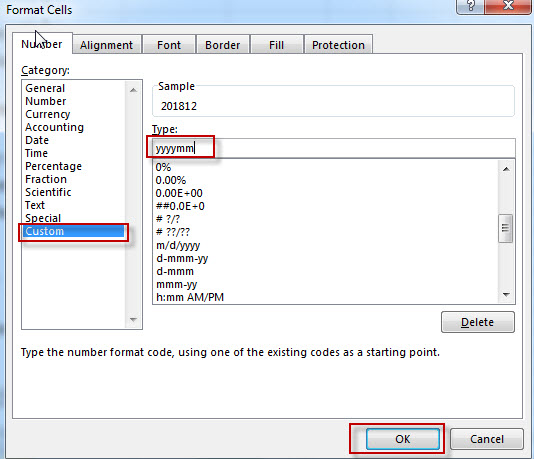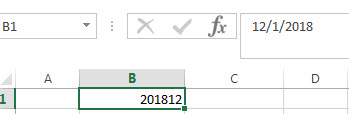# Convert date to month and year only in excel

In excel, how to get the month and year string from an excel date value. How to convert the dates including both date and time to text string, just only display as year and month. This post will guide you how to convert date to month and year value using Text Function in Microsoft Excel.

The Excel TEXT function converts a numeric value into text string with a specified format. You can convert all the standard number formats such as: dates, times, currency to Text string in excel.

The syntax of the TEXT function is as below:

`= TEXT (value, Format code)`

## Convert date to month and year with Text Function

If you want to convert the date to month and year only, you can use “yyyymm” format code within the TEXT function in excel, so you can write down the below TEXT formula:

`=TEXT(date,"yyyymm")`Assuming that you want to get the text string of month and year only from the date value: 12/1/2018 in Cell B1, then you can enter the following TEXT function into Cell C1.

In the above example, the TEXT function will apply the format code that you specified and returns a text string “201812” in Cell C1.

For more format codes in excel formatting, you can refer to the below table:

 Format Code Description Examples 0 only display digits in its place #.00 – Forces the function to display two decimal places =Text(34.234,”\$##.00″) result: \$34.23 # Display the placeholder =Text(4.527,”#.##) result: 4.53 . the position of Decimal Point =Text(342.2,”0.00″) result: 342.20 d Day of the month or day of week d- one or two digit number (1-31) dd- two digit number (01-31) ddd-abbreviated day of week (Mon to Sun) dddd-full name of day of week(Monday to Sunnday) =Text(TODAY(),”DDDD”) result: Monday m The Month of the Year m- one or two digit number mm-two digit number mmm-abbreviated month(Jan to Dec) mmmm-full name of month(January to December)) =Text(TODAY(),”MM/DD/YY”) result:11/06/17 y year yy-two digit representation of year(e.g.01,17) yyyy-four digit representation of year(e.g. 2001,2017) =Text(TODAY(),”MM/DD/YY”) result:11/06/17 h Hour h-one or two digit number (e.g. 1,23) hh-two digit number (e.g. 01,23) =Text(14:16,”hh:mm”) result: 14:16 m Minute m-one or two digit representation (e.g. 1,59) mm-two digit representation (e.g. 01,59) =Text(14:16,”hh:mm”) result: 14:16 s Second s-one or two digit representation (e.g. 1,59) ss=two digit representation (e.g. 01,59)

## Convert date to month and year with Format Cell option

If you just want to display a date from 12/1/2018 to “201812” in cell B1, you do not need a formula, just refer to the following steps:

1# selected the cells you want to display a date with the year and month.2# right click on the selected cells and click on “Format cells…”

3# Select “Custom” under “Number” tab, then type the custom format “yyyymm”in type.4# you will see that the date value is converted to month and year.### Related Formulas

• Excel Convert numbers to Text
The Text function will accept a numeric value as the first argument, then based on the format code in the second argument to convert the number to text. You can convert all the standard number formats such as: dates, times, currency to Text string in excel.…
• Convert date to month name with Text Function
If you want to convert the date to a month name, you can use the TEXT function with a specified format code like “mmm”in excel.  You can try to run the following TEXT formula:=TEXT(B1,”mmm”)
• Convert Date to text with Text Function in Excel
you can use TEXT function in excel to convert dates to text in a specific format code. For example, The TEXT function can use the following patterns, like as: “mm/dd/yyyy”, “yyyy/mm/dd”, etc.

### Related Functions

• Excel Text function
The Excel TEXT function converts a numeric value into text string with a specified format. The TEXT function is a build-in function in Microsoft Excel and it is categorized as a Text Function. The syntax of the TEXT function is as below: = TEXT (value, Format code)…
Related Posts

How to apply a formula in entire column or row without/with dragging

This post explains that how to apply an excel formula in entire column or row through dragging fill handler in excel. How do I apply formula in entire column or row without dragging fill handler by shortcut or Fill command. ...

Get the Current Workbook Name

This post explains how to get the current workbook name in excel. Get the Current Workbook Name In excel, the CELL function can get the information of the current worksheet, it will return the full file name and its path. ...

Split Text and Numbers in Excel

This post explains how to split text and numbers in excel, or how to extract the text characters from another strings with text and numbers combined. Split Text and Numbers If you want to split text and numbers, you can ...

Split Text String by Specified Character in Excel

This post explains how to split text string by a specified character (such as: comma, space, dash, slash, etc.) using FIND function within other functions in excel. And you will learn that how to extract part of characters to the ...

Excel Replace Function Remove Text String

This post will teach you how to remove a text string of specified length from another text string in a cell. Excel Replace Function Remove Text String If you want to remove text string from a cell in excel, you ...

Combining the REPLACE Function with FIND Function to Remove Text in Excel

How to remove a text string or characters from a cell when the text is at a specific position. And this post will guide you how to remove a text string from a cell or column that the removed text ...

Combining the Replace function with Text function in Excel

In excel, we can use Replace function in combination with Text function to replace date characters that are part of the text string. And this post will guide you how to use TEXT function as the first arguments to replace ...

Excel Replace function with numeric values

In excel, how to replace a numeric characters within a text string using another numeric characters. This post will guide you how to use REPLACE function to replace numeric characters using another numeric characters in one text string. The Excel ...

Convert Date to Day of Week in Excel (get day name from date )

In excel, how to get the day of week from an excel date value. How to return a day name of the week from a date that is contained in a cell in Microsoft Excel. This post will guide you ...

Convert date to month and day only in excel

In excel, how to get the month and day string from an excel date value. How to convert the dates to text string, just only display as month and day. This post will guide you how to convert date to ...

Sidebar